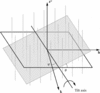International
Tables for
Crystallography
Volume F
Crystallography of biological macromolecules
Edited by M. G. Rossmann and E. Arnold

International Tables for Crystallography (2006). Vol. F, ch. 19.2, pp. 425-426   | 1 | 2 |

## Section 19.2.4.1. Data sampling

W. Chiua*

aVerna and Marrs McLean Department of Biochemistry and Molecular Biology, Baylor College of Medicine, Houston, Texas 77030, USA
Correspondence e-mail: wah@bcm.tmc.edu

#### 19.2.4.1. Data sampling

| top | pdf |

The principle of three-dimensional reconstruction is based on the central section theorem, which states that the experimental or computed projected diffraction pattern of a three-dimensional object is a plane that intersects the centre of the three-dimensional Fourier space in the direction normal to the direction of the projection (DeRosier & Klug, 1968). Because of the crystallographic symmetry inherent in a protein crystal, only a portion of the entire three-dimensional Fourier space, equivalent to an asymmetric unit of the crystal unit cell, is needed for the reconstruction. The structure factors of a three-dimensional crystal are localized in the three-dimensional reciprocal lattice, whereas the structure factors of a two-dimensional crystal are distributed continuously along the lattice lines, each of which passes through the reciprocal lattice in the zero projection plane (Fig. 19.2.4.1)(Henderson & Unwin, 1975). The assignment offor each observation (h, k,) along the lattice line is determined from the tilt angle and direction of the tilt axis for each image (Shaw & Hills, 1981). In general, the three-dimensional data set is initially built up from low-angle data and is gradually extended to the high-angle data. The angular parameters for each observed reflection are iteratively refined among one another within the whole data set. The required accuracy of these angular determinations depends on the thickness of the crystal and also on the desired resolution (Prasad et al., 1990). For instance, the angular accuracy has to be < 0.1° for 3.5 Å data in a 100 Å-thick crystal. The sampling of the data along the lattice line is generally aboutthe thickness of the crystal (Henderson et al., 1990). The data points are not evenly sampled along the lattice lines; they must be fitted into continuous and smoothly varying functions within the constraint of the crystal thickness. These functions are interpolated onto a periodic lattice, so that its inverse Fourier transform can be computed to reconstruct the three-dimensional mass-density function of the object.Figure 19.2.4.1 | top | pdf |Schematic diagram of data distribution in Fourier space for a two-dimensional crystal. Both the amplitudes and phases of the structure factors are distributed along the lattice lines passing through the (h, k) projection plane. The sectional plane shown denotes data from a crystal tilted at a certain angle (ϕ), and its tilt axis has an inclined angle (θ) with respect to one of its crystallographic axes. (Courtesy of Dr Jaap Brink at Baylor College of Medicine.)

### References

DeRosier, D. J. & Klug, A. (1968). Reconstruction of three-dimensional structures from electron micrographs. Nature (London), 217, 130–134.Google Scholar
Henderson, R., Baldwin, J. M., Ceska, T. A., Zemlin, F., Beckmann, E. & Downing, K. H. (1990). Model for the structure of bacteriorhodopsin based on high-resolution electron cryo-microscopy. J. Mol. Biol. 213, 899–929.Google Scholar
Henderson, R. & Unwin, P. N. (1975). Three-dimensional model of purple membrane obtained by electron microscopy. Nature (London), 257, 28–32.Google Scholar
Prasad, B. V., Degn, L. L., Jeng, T. W. & Chiu, W. (1990). Estimation of allowable errors for tilt parameter determination in protein electron crystallography. Ultramicroscopy, 33, 281–285.Google Scholar
Shaw, P. J. & Hills, G. J. (1981). Tilted specimen in the electron microscope: a simple specimen holder and the calculation of tilt angles for crystalline specimens. Micron, 12, 279–282.Google Scholar